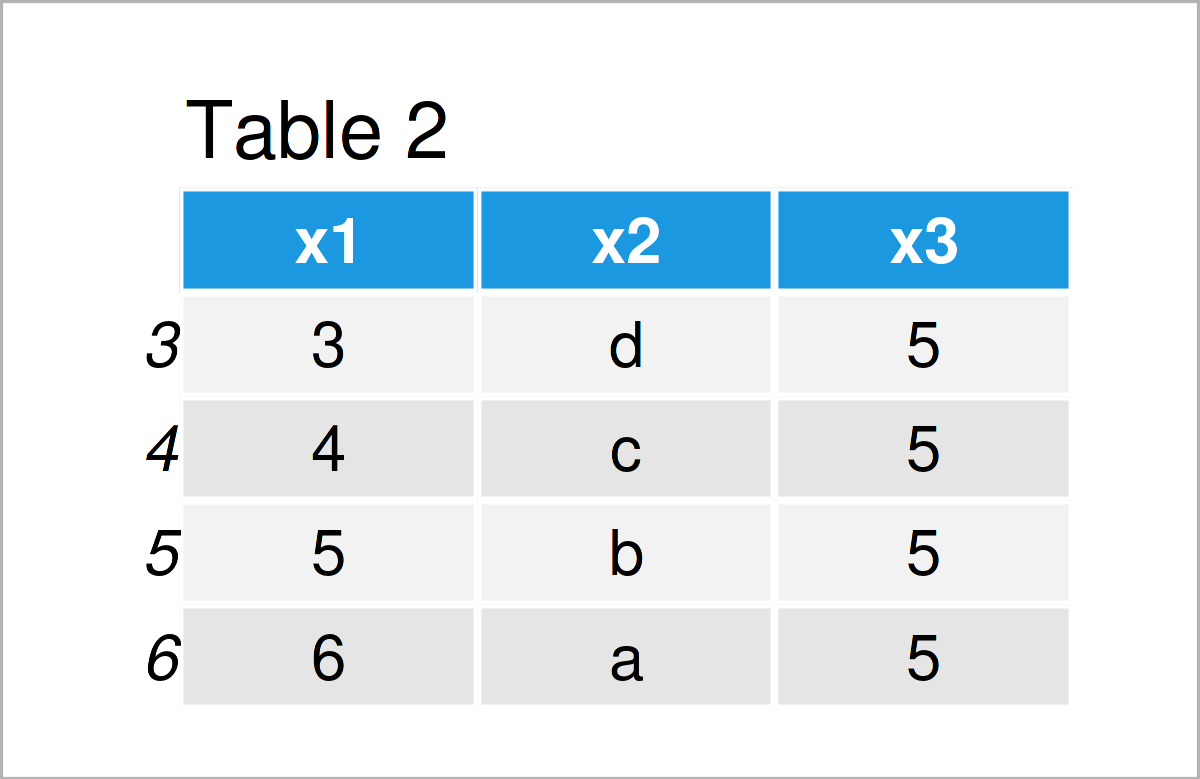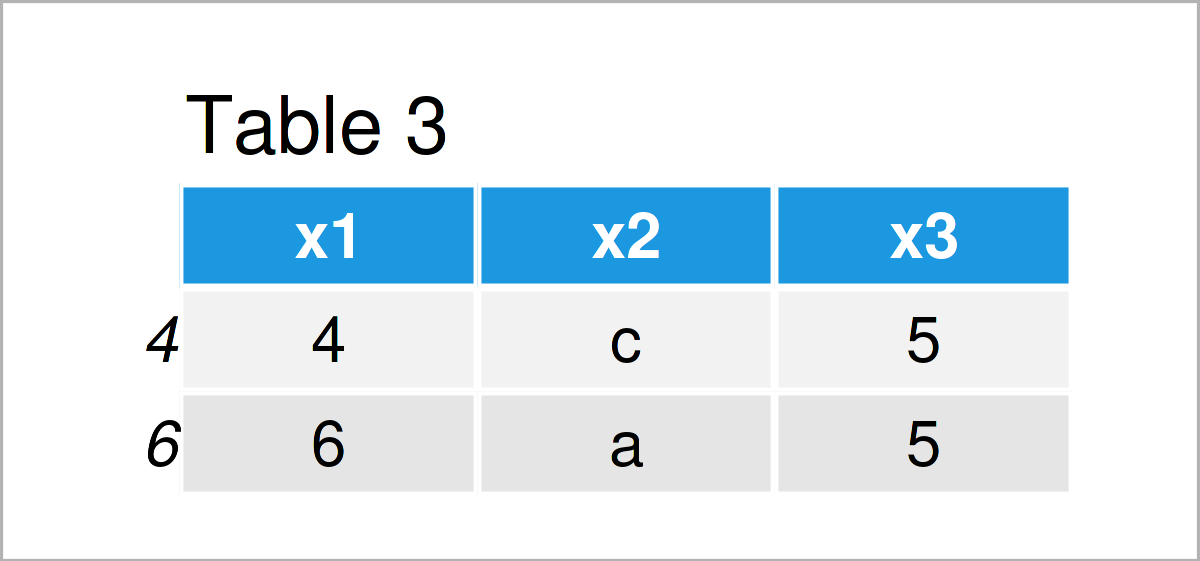# which Function & Data Frame in R (2 Examples)

In this article, I’ll explain how to apply the which function to a data frame in the R programming language.

You’re here for the answer, so let’s get straight to the exemplifying R code!

## Creation of Example Data

Have a look at the following example data frame:

```data <- data.frame(x1 = 1:6,                        # Create example data frame
x2 = letters[6:1],
x3 = 5)
data                                                # Print example data frame```As you can see based on Table 1, our example data is a data frame constructed of six rows and three columns.

## Example 1: Apply which() Function to Create Data Frame Subset

This example shows how to use the which function to extract certain rows from our data frame based on a logical condition.

Consider the R code below:

```data_new1 <- data[which(data\$x1 >= 3), ]            # Apply which function
data_new1                                           # Print data frame subset```By executing the previous code, we have created Table 2, i.e. a new data frame object containing only those rows where the value in the column x1 is greater or equal to 3.

## Example 2: Using Multiple Logical Conditions within which() Function

In Example 2, I’ll demonstrate how to use multiple logical conditions within the which function to subset a data frame.

For this task, we can use the & operator as shown below:

```data_new2 <- data[which(data\$x1 >= 3 & data\$x2 %in% c("a", "c", "e")), ]  # Apply which()
data_new2                                           # Print data frame subset```The output of the previous syntax is shown in Table 3 – A data frame subset based on multiple logical conditions.

## Video & Further Resources

In case you need further explanations on the R codes of this article, I recommend having a look at the following video on my YouTube channel. In the video, I explain the examples of this tutorial in a live session.

Please accept YouTube cookies to play this video. By accepting you will be accessing content from YouTube, a service provided by an external third party.If you accept this notice, your choice will be saved and the page will refresh.

Furthermore, you may want to have a look at some related articles on my homepage:

On this page you have learned how to apply the which function to the rows of a data frame in R. If you have any further questions, let me know in the comments section.

Subscribe to the Statistics Globe Newsletter